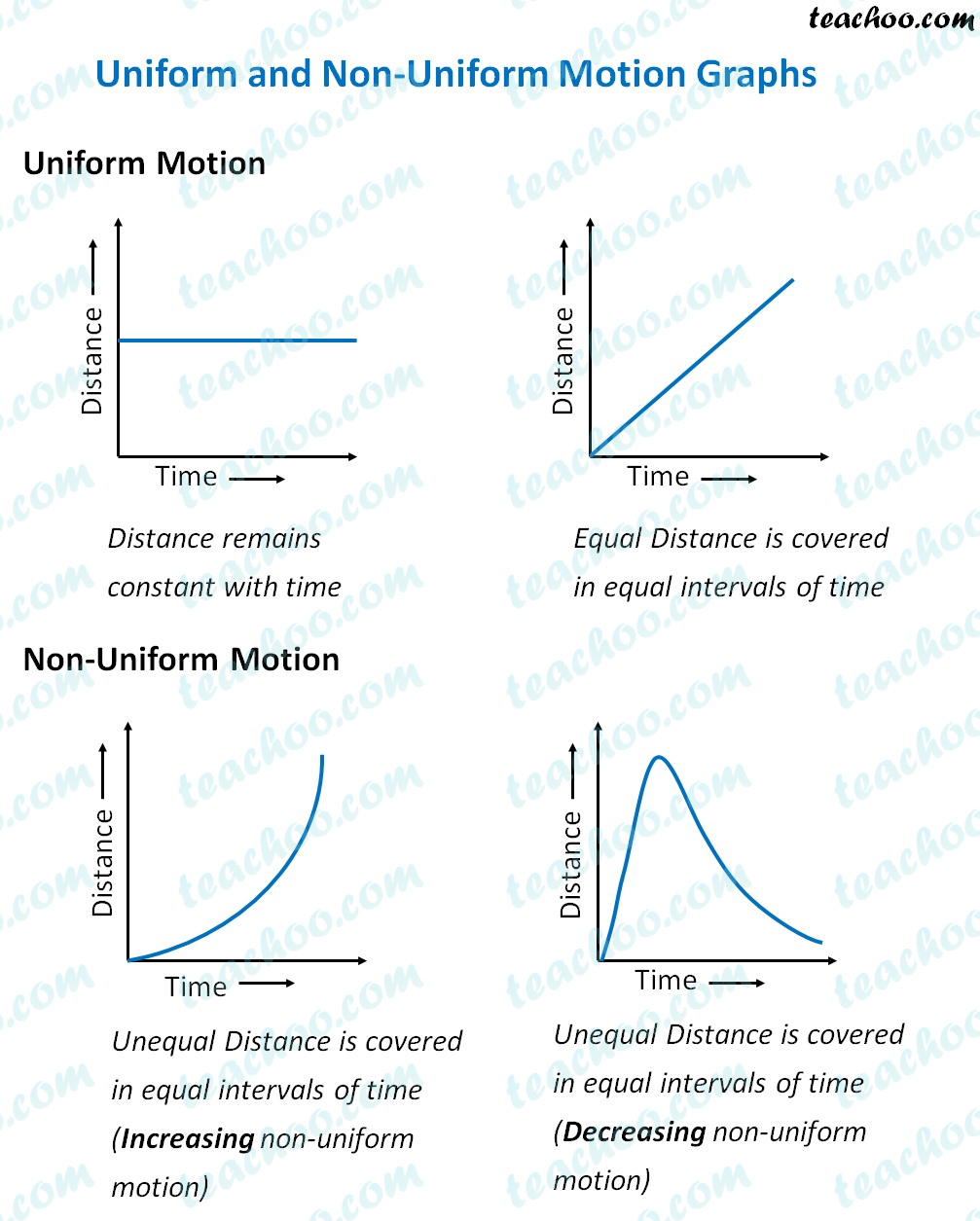Concepts

Class 9
Chapter 8 Class 9 - Motion

Suppose there are 2 cars

Car 1 and Car 2

Both Car cover a distance of 80 km

Car 1 Covers 40 km in first hour and 40 Km in second hour

Car 2 Covers 30 km in First Hour and 50 Km In Second Hour

It is said that

Car 1 is in Uniform Motion

Car 2 is in Non Uniform Motion

## What is Uniform Motion?

A body is in uniform motion if it covers equal distance in equal intervals of time

Example

Car 1 travelling at 40 km in both first and second hour

## What is Non Uniform Motion?

A body is in non uniform motion if it covers unequal distance in equal intervals of time

Example

Car 1 travelling at 30 km in first hour and 50 km in second hour

Note

Non Uniform Motion is also called Accelerated MotionNote
Graph of Uniform Motion is Straight Line

Graph of Non - Uniform Motion is Curved Line

#### In Uniform Motion, Equal Distance is Traveled even over Small Distances

Note

If a body travels at a uniform speed,it means it travels equal distance at equal intervals of time.

It does not matter how small these intervals of time are.

Example

Suppose a train travels 120 kilometer in 1 Hour in uniform motion

It means ,it travels at a uniform speed

In 60 Minutes = 120 Km

In 1 Minutes = 120/60 = 2 Km

In 60 Seconds = 2000 m

I 1 Second = 2000/60 = 33.33 meter

## Difference between uniform and non-uniform motion

 Uniform Motion Non Uniform Motion It has zero acceleration. It has non-zero acceleration. Distance-time graph shows a straight line Distance-time graph shows a curved line Covers equal distances in equal time interval. Covers unequal distances in equal time interval. Its average speed is same as speed of the object Its average speed is different as speed of the object

## Questions

Q 4 Page 102 - What does the path of an object look like when it is in uniform motion?

Learn in your speed, with individual attention - Teachoo Maths 1-on-1 Class